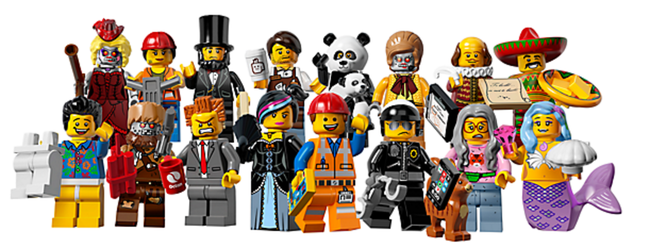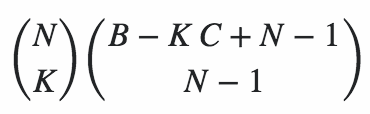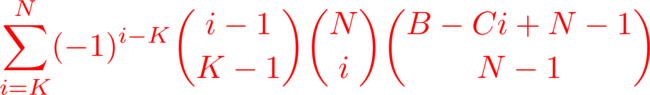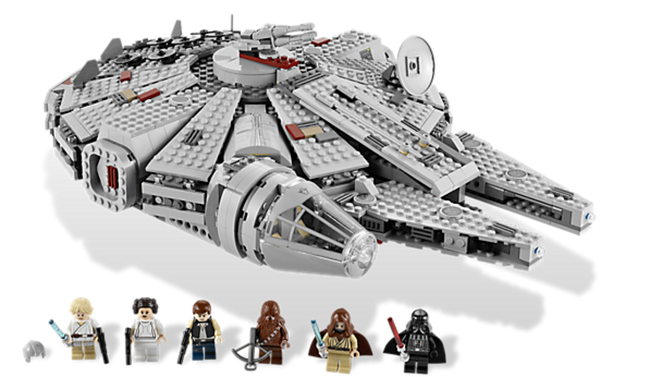# General Collector’s Problem

Some time ago at work we wanted a way to represent each person with an avatar to track what they were working on on a board. We were using a sort of Kanban in software engineering and I though it might be fun to get some LEGO mini-figures to represent each person in the team on the board. I was wondering it would be cheaper to just buy a complete set of say 8 LEGO mini-figures at a premium. Or instead buy a number of random individual packs in the hope of getting a set of unique figures without spending all the money on the full set.I did some reading and found that this is called the coupon collector’s problem problem which seeks to work out the expected number of purchases that need to be made before we have a complete set. In the case of an `8` figure set the expected number of purchases is `22`. That is to say that you should expect to purchase `22` random figures before you have at least one of each of the 8 possible figures. Say a single figure cost `\$10` where as the complete set cost `\$130` it makes sense to buy the complete set. It’s both cheaper and less riskier. But what if we only want five unique figures from the set of `8`. In this case there is a probability that you will get `5` uniques and that probability grows the more random figures you purchase and ultimately converges to `100%` as you purchase more figures. For example there is a `89.4%` chance that you will get five unique figures if make `9` purchases which will cost `\$90`.

Number of purchases Probability of 5 unique figures
0,1,2,3,4 0.0
5 0.188
6 0.471
7 0.679
8 0.807
9 0.894
10 0.938

In general we can compute the expected number of purchases directly. Let’s say we are starting out our purchasing run and are trying to compute the expected number of purchases `E(X)` required to get `X` remaining different figures from `N` possible available figures. There are two possible outcomes from any given purchase. Our new figure is a duplicate of one we already have, or it isn’t.

1. If it’s a duplicate we are in exactly the same position except that we have made an extra purchase. The probability of this happening is: `P = 1/X` so `E(X) = P * (1 + E(X))`

2. It it’s not a duplicate we have a new figure in our collection and there are now fewer outstanding figures to collect. The probability of this happening is: `1 - P`. Therefore `E(X) = (1 - P) * E(X-1)`.

Adding these equations and solving for `E(X)` gives `E(X) = N * (1/1 + 1/2 + ... + 1/N)` which we can compute with:

``````def harmonic(x):
return sum( 1.0 / float(i) for i in xrange(1, x+1))

N = 8
print N * harmonic(N) # 21.742
``````

or for larger values, we can approximate it pretty closely with:

``````import numpy
def approximate(x):
gamma = 0.5772156649
return x*numpy.log(x) + gamma*x + 0.5

N = 8
print approximate(N) # 21.753
``````

The complication came in where we wanted to have `C` copies of `K` different figures. Because people could be working on more than one task. For example `C = 2` and `K = 2` means we wanted duplicates of at least two different figures. This seems to be a generalization of the coupon collector’s problem to a situation where we want to make `B` purchases, each will be one of `N` different uniformly random figures. And we would like to know the probability that after these `B` purchase we have at least `C` copies of `K` different figures. The original problem we looked as was the same at this with `N = 8`, `C = 1`, `K = 5` for increasing values of `B`.

Let’s look at the case of `B = 5`, `N = 3`, `C = 2`, `K = 2`. Here there are three different types of figures, lets call them `X1`, `X2` and `X3`. We are making `B = 5` purchases. We could get `X1, X1, X1, X2, X2` which would be a success. So would getting `X1, X1, X2, X3, X3`. However `X1, X2, X3, X3, X3` would not because we only have more than `2` copies of the `X3` figure where as we only have `1` copy of the `X1` and `X2` figures. We can’t simply track the number of unique figures we have and deduce the number we don’t have as we did for computing the expectation for the coupon collector’s problem. We need to know the number we have of each type of figure.

Counting the number of different ways we can make successful purchases is the same as counting the number of non-negative integer solution to the equation: `X1 + X2 + X3 = B` with the additional constraint that at least `K` of the variables should be greater than or equal to `C`. Without constraints the number of solutions is `B + 1` multichoose `K - 1` which is the same and `B + K - 1` choose `B`. In order to solve it with the extra constraints we can assume that the first `K` variables are the ones that are going have counts of at least `C` and make change of variable to `Yi = Xi - C` for the first `K` variables. Then we want to count the number of non-negative integer solutions to
`Y1 + Y2 + X3 = B - CK` instead. And multiply this by the number of ways to select the subset of `K` variables of which we will have at least `C` copies. The number of solutions is again `B − KC + N − 1` choose `N − 1` and the number of ways of selecting the `K` variables is `N` choose `K` which gives this beast:However this will actually double count some purchases when `B − KC < C`. Because then we have enough excess purchase to buy `C` copies of a figure which already be counted as one of the solutions to `Y1 + Y2 + X3 = B - CK`. To fix this we need to remove the double counted solution using the Inclusion-exclusion principle which finally gives us this expression for the number of groups of purchases:We still need to count the number of permutations of these groups of purchases to get the final solution and I’m still on the hunt for that. In the mean time compute the probability of can be done by enumerating successfully possibility and dividing by the total number of outcomes `N**K`

``````def simulate(sample, B, N, C, K):

if len(sample) == B:
counts =  * N
for s in sample: counts[s] += 1
success = filter(lambda h: h >= C, counts)
success = len(success) >= K
return int(success)

solutions = 0
for i in xrange(N):
sample.append(i)
solutions += simulate(sample, B, N, C, K)
sample.pop()
return solutions

if __name__ == '__main__':
B, N, C, K = 10, 8, 2, 2
sample = []
Z = simulate(sample, B, N, C, K)
print 'Probability: ', float(Z) / float(N**B)
``````

Which gives the follow probabilities of getting at least two copies of two different figures:

Number of purchases Probability of two duplicates
0,1,2,3 0.0
4 0.041
5 0.170
6 0.389
7 0.635
8 0.828
9 0.937

In the end we didn’t actually end up doing either of these things and instead got the LEGO Millennium Falcon which seemed like a much better decision because then you get Darth Vader and also the top opens and you can see inside. Thanks to all these great peeps for helping me out on this.